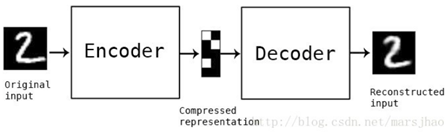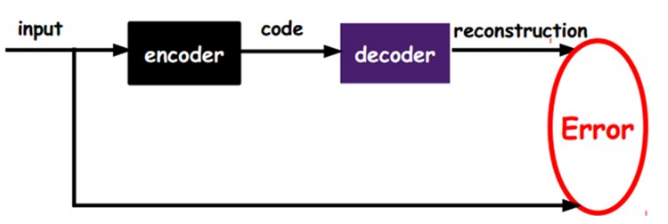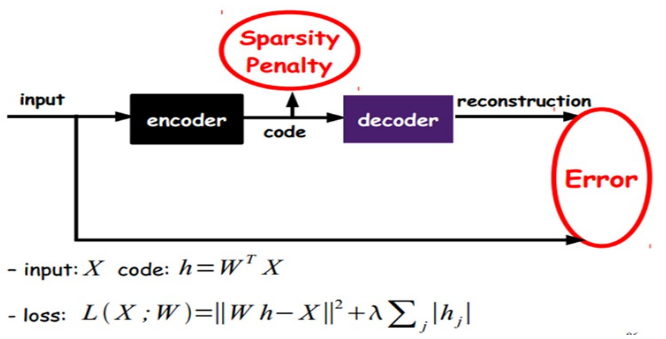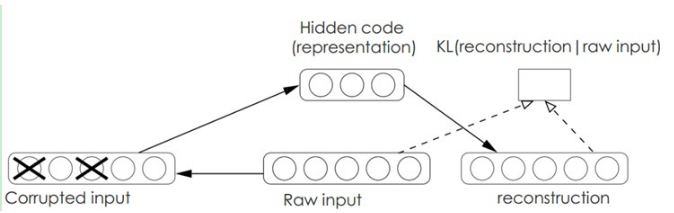# 深度学习之卷积自编码器

1. 编码器：将输入压缩为潜在空间表示。可以用编码函数h = f（x）表示。
2. 解码器：这部分旨在重构来自隐藏空间表示的输入。可以用解码函数r = g（h）表示。因此整个网络可以看成是要g(f(x))和x尽量接近。具体操作如下图，我们将input输入一个encoder编码器，就会得到一个code，这个code也就是输入的一个表示，然后加一个decoder解码器，这时候decoder就会输出一个信息，那么如果输出的这个信息和一开始的输入信号input是很像的（理想情况下就是一样的），那很明显，我们就有理由相信这个code是靠谱的。所以，我们就通过调整encoder和decoder的参数，使得重构误差最小，这时候我们就得到了输入input信号的第一个表示了，也就是编码code了。因为是无标签数据，所以误差的来源就是直接重构后与原输入相比得到。1）自动编码器是数据相关的（data-specific 或 data-dependent），这意味着自动编码器只能压缩那些与训练数据类似的数据。比如，使用人脸训练出来的自动编码器在压缩别的图片，比如树木时性能很差，因为它学习到的特征是与人脸相关的。

2）自动编码器是有损的，意思是解压缩的输出与原来的输入相比是退化的，MP3，JPEG等压缩算法也是如此。这与无损压缩算法不同。

3）自动编码器是从数据样本中自动学习的，这意味着很容易对指定类的输入训练出一种特定的编码器，而不需要完成任何新工作。

1、卷积自编码器

# ENCODER
conv_1 = tf.layers.conv2d(x_input, 32, (4, 4), strides=(1, 1), padding='valid',
kernel_initializer=tf.truncated_normal_initializer)  # => (142, 142, 32)
batch_norm_1 = tf.nn.relu(tf.layers.batch_normalization(conv_1, training=is_training))   # (142, 142, 32)
conv_2 = tf.layers.conv2d(batch_norm_1, 64, (3, 3), strides=(1, 1), padding='valid',
kernel_initializer=tf.truncated_normal_initializer)  # =>(140, 140, 64)
batch_norm_2 = tf.nn.relu(tf.layers.batch_normalization(conv_2, training=is_training))  # (140, 140, 64)
pooling_1 = tf.layers.max_pooling2d(batch_norm_2, pool_size=(5, 5), strides=(5, 5))   # =>(28, 28, 64)
conv_3 = tf.layers.conv2d(pooling_1, 128, (3, 3), strides=(1, 1), padding='valid',
kernel_initializer=tf.truncated_normal_initializer)   # =>(26, 26, 128)
batch_norm_3 = tf.nn.relu(tf.layers.batch_normalization(conv_3, training=is_training))    # (26, 26, 128)
pooling_2 = tf.layers.max_pooling2d(batch_norm_3, pool_size=(2, 2), strides=(2, 2))   # =>(13, 13, 128)
self.code = pooling_2
# DECODER
conv_trans_1 = tf.layers.conv2d_transpose(pooling_2, 128, kernel_size=(2, 2), strides=(2, 2))  # =>(26, 26, 128)
conv_trans_1 = tf.nn.relu(tf.layers.batch_normalization(conv_trans_1, training=is_training))  # (26, 26, 256)

conv_trans_2 = tf.layers.conv2d_transpose(conv_trans_1, 64, kernel_size=(3, 3), strides=(1, 1)) # =>(28, 28, 64)
conv_trans_2 = tf.nn.relu(tf.layers.batch_normalization(conv_trans_2, training=is_training))  # (28, 28, 128)

conv_trans_3 = tf.layers.conv2d_transpose(conv_trans_2, 64, kernel_size=(5, 5), strides=(5, 5))  # =>(140, 140, 64)
conv_trans_3 = tf.nn.relu(tf.layers.batch_normalization(conv_trans_3, training=is_training))  # (140, 140, 128)

conv_trans_4 = tf.layers.conv2d_transpose(conv_trans_3, 32, kernel_size=(3, 3), strides=(1, 1))  # (142, 142, 32)
conv_trans_4 = tf.nn.relu(tf.layers.batch_normalization(conv_trans_4, axis=3))  # (142, 142, 64)

conv_trans_5 = tf.layers.conv2d_transpose(conv_trans_4, 1, kernel_size=(4, 4), strides=(1, 1))  # (145, 145, 32)
conv_trans_5 = tf.nn.relu(tf.layers.batch_normalization(conv_trans_5, axis=3))  # (145, 145, 2)

conv_final = tf.layers.conv2d(conv_trans_5, 1, kernel_size=(2, 2), strides=(1, 1), padding='same')  #(145, 145, 1)



如果隐藏节点比可视节点（输入、输出）少的话，由于被迫的降维，自编码器会自动习得训练样本的特征（变化最大，信息量最多的维度）。但是如果隐藏节点数目过多，甚至比可视节点数目还多的时候，自编码器不仅会丧失这种能力，更可能会习得一种“恒等函数”——直接把输入复制过去作为输出。这时候，我们需要对隐藏节点进行稀疏性限制。所谓稀疏性，就是对一对输入图像，隐藏节点中被激活的节点数（输出接近1）远远小于被抑制的节点数目（输出接近0）。那么使得神经元大部分的时间都是被抑制的限制则被称作稀疏性限制。3、降噪自编码器

降噪自动编码器DA是在自动编码器的基础上，训练数据加入噪声，所以自动编码器必须学习去去除这种噪声而获得真正的没有被噪声污染过的输入。因此，这就迫使编码器去学习输入信号的更加鲁棒的表达，这也是它的泛化能力比一般编码器强的原因。DA可以通过梯度下降算法去训练。http://www.atyun.com/16921.html

https://blog.csdn.net/u010555688/article/details/24438311

​​​​​​